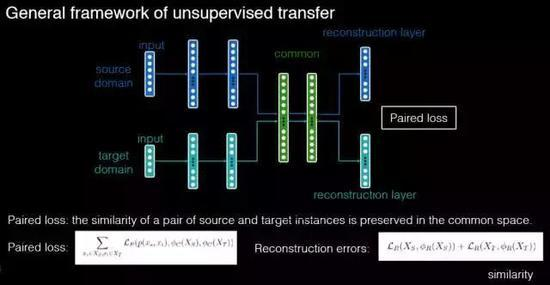Covariate Shift Based：

 James Heckman. Sample Selection Bias as a Specification Error. 1979. Nobel prize-winning paper which introduced Sample Selection Bias.

 Arthur Gretton et al. Covariate Shift by Kernel Mean Matching. 2008. Describes a procedure for computing the optimal source weights, given infinite unlabeled data and a universal kernel. Also provides target error bounds when learning under the labeled source distribution.

 Corinna Cortes et al. Sample Selection Bias Correction Theory. 2008. Analyzes the case when we have only finite unlabeled data, so that we cannot determine the optimal weights exactly. This leads to a bias when learning from

the source domain.

 Steffen Bickel. Discriminative Learning under Covariate Shift. 2009. Introduces the logistic regression model for learning source weights directly from unlabeled data.

Representation Learning Based：

 John Blitzer et al. Domain Adaptation with Structural Correspondence Learning. 2006. Describes the projection-learning technique from this section.

 Shai Ben-David et al. Analysis of Representations for Domain Adaptation. 2007. Gives an early version of the discrepancy distance and its relation to adaptation error.

 John Blitzer et al. Domain Adaptation for Sentiment Classification. 2007. Describes an application of projection learning to sentiment classification. These are the results from the previous section.

 Yishay Mansour et al. Domain Adaptation: Learning Bounds and Algorithms. 2009. Generalizes the discrepancy distance to arbitrary losses and gives a tighter bound than above. Also describes techniques for learning instances weights to directly minimize discrepancy distance.

 T. Evgeniou and M. Pontil. Regularized Multi-task Learning. 2004.

 Hal Daume III. Frustratingly Easy Domain Adaptation. 2007. Describes the feature replication algorithm from this section.

 Kilian Weinberger et al. Feature Hashing for Large Scale Multitask Learning. 2009. Describes the feature hashing technique for handling millions of domains or tasks simultaneously.

 A. Kumar et al. Frustratingly Easy Semi-supervised Domain Adaptation. 2010.Shows how to incorporate unlabeled data in the feature replication framework.

 Olivier Chapelle et al. A machine learning approach to conjoint analysis. 2005.

 Kai Yu et al. Learning Gaussian Processes from Multiple Tasks. 2005. Kernelized multi-task learning with parameters linked via GP prior.

 Ya Xue et al. Multi-task Learning for Classification with Dirichlet Process Priors. 2007. Learning with parameters linked via DP clustering.

 Hal Daume III. Bayesian Multitask Learning with Latent Hierarchies. 2009. Describes the latent hierarchical modeling.

<纯干货-5>Deep Reinforcement Learning深度强化学习_论文大集合
<模型汇总-9> VAE基础：LVM、MAP、EM、MCMC、Variational Inference（VI）
<模型汇总-6>基于CNN的Seq2Seq模型-Convolutional Sequence to Sequence